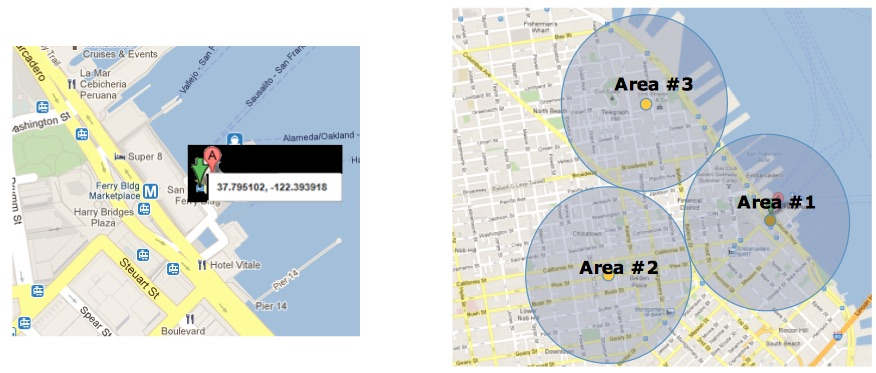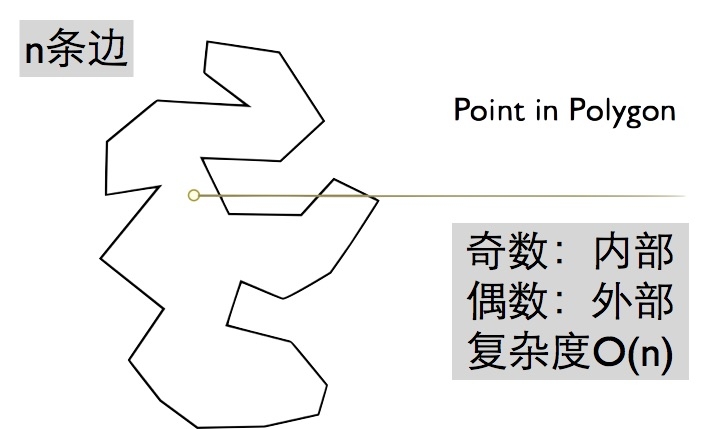# 车联网 - 电子围栏概述

## 工作流程1. 车载设备需要安装GPS设备，并能实时定位当前位置主动上报。
2. 管理者在TSP系统中设定车辆行驶在哪一个指定区域里，并制定出当车辆驶出指定区域后的解决方案。
3. 车辆通过4G/5G通讯网络传输当前车辆位置信息TSP系统，系统存储车辆w位置数据和并进行实时分析，记录和备份车辆的行驶路径。
4. 如果车辆驶出设定的指定区域，TSP系统则推送警报给管理者以告知。

## 电子围栏算法

#### 1. 射线法#### 2. 夹角和法## 代码示例

``````import json
import math
lnglatlist = []

data = '[{"points":[{"lng":0,"lat":0},{"lng":2,"lat":0},{"lng":0,"lat":2}]}]'

if 'points' in data:
for point in data['points']:
lnglat = []
lnglat.append(float(str(point['lng'])))
lnglat.append(float(str(point['lat'])))
lnglatlist.append(lnglat)

def windingNumber(point, poly):
poly.append(poly)
px = point
py = point
sum = 0
length = len(poly)-1

for index in range(0,length):
sx = poly[index]
sy = poly[index]
tx = poly[index+1]
ty = poly[index+1]

#点与多边形顶点重合或在多边形的边上
if((sx - px) * (px - tx) >= 0 and (sy - py) * (py - ty) >= 0 and (px - sx) * (ty - sy) == (py - sy) * (tx - sx)):
return "on"
#点与相邻顶点连线的夹角
angle = math.atan2(sy - py, sx - px) - math.atan2(ty - py, tx - px)
#确保夹角不超出取值范围（-π 到 π）
if(angle >= math.pi):
angle = angle - math.pi * 2
elif(angle <= -math.pi):
angle = angle + math.pi * 2
#计算回转数并判断点和多边形的几何关系
result = 'out' if int(sum / math.pi) == 0 else 'in'
return result

point = [1, 1]
print(windingNumber(point,lnglatlist))

``````